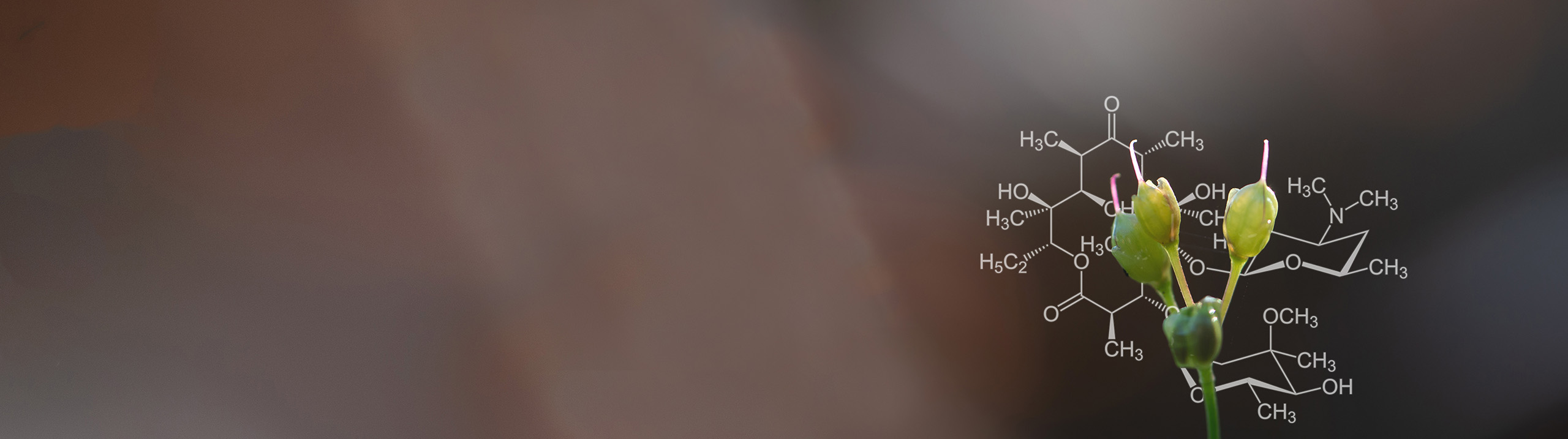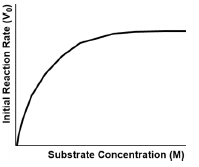Start typing, then use the up and down aroows to select an option from the list.# Biochemistry

Learn the toughest concepts covered in Biochemistry with step-by-step video tutorials and practice problems by world-class tutors

6. Enzymes and Enzyme Kinetics
1

### concept

Initial Velocity8m
Play a video:
0
2

### concept

Initial Velocity9m
Play a video:
0
3
Problem

Why is the initial velocity (V0) the best chance a reaction has at approaching its maximum velocity (Vmax)?

4
Problem

Calculate the initial reaction rate for A → B, given that [A] i = 9.6 M, [B] i = 0 & [A] f after 0.01 μsec = 9.14 mM.

5
Problem

Imagine that you’re setting up an experiment to measure the kinetics of an enzyme. Which option below indicate reasons for why it’s important to measure the enzyme’s initial velocity immediately after starting the reaction?

6
Problem

In the graph below, why does the curve have a steep incline at first that gradually declines to a horizontal line?